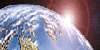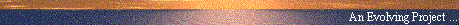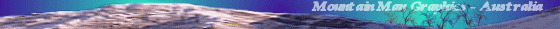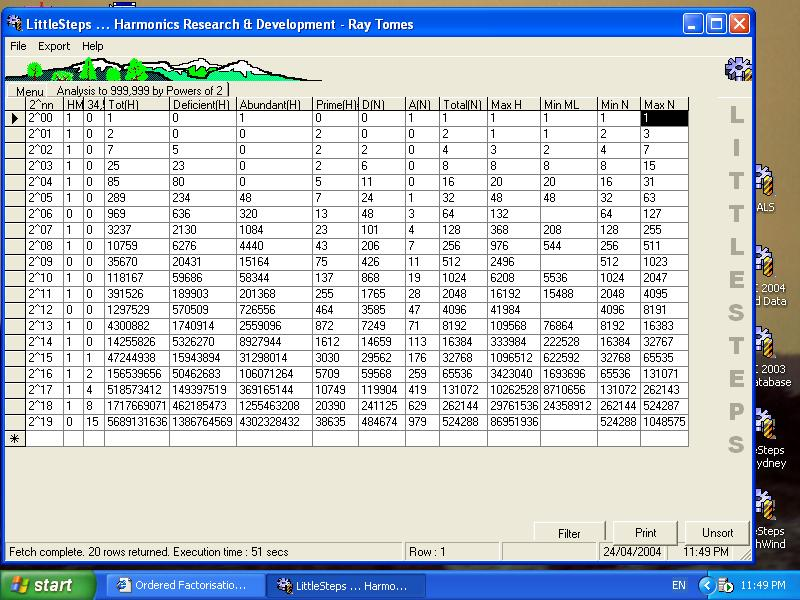# Number of Ordered Factorizations

#### Primitive computational analyses employing the ancient parameters of deficient and abundant regarding the number of ordered factorizations. PRF Brown Falls Creek, Southern Autumn of 2004

##### Web Publication by Mountain Man Graphics, Australia## Introduction

The following work represents a small amount of computational research concerning the nature of ordered factorizations, and specifically the number of ordered factorizations H(n) for any given n. To obtain a brief outline of the subject matter, which will be assumed in this article, please see the reference index page, and the listed resource and definition information.

The first section deals with the ability of the number of ordered factorization to account for the harmonic relationships evident in the musical Just Intonation Scale, and introduces the notion of aliquot type.

The second section examines the ancient notion of aliquot type historically (Euclid) defined by summation of the component aliquots, and notes the three separate threads of number so formalised. Abundant numbers have the sum of their divisors greater than themselves, deficient numbers have the sum of their divisors less than themselves. In the middle, with the sum of their divisors exactly equal to the number are the "Perfect Numbers".

The issue of Perfect Numbers (see above reference) and their relationship to Mersenne Primes has recently been popularised in distributed computational arrangments over the internet. For the latest in prime technology visit www.mersenne.org.

The third section introduces a new application for these ancient aliquot types. A redefinition in terms of the number of ordered factorisations (divisors) for any given number (rather than the summation of the divisors) is investigated.

That is, we define the following categories in regard to the number of ordered factorizations.
Let the number of ordered factors of n be H(n), then:

• Deficient Ordered Factorizations ... H(n) < n
Note that this set includes the primes for which by definition H(n) = 1.

• Balanced Ordered Factorizations ... H(n) = n
The term balanced was used in preference to "perfect".
The first six balanced numbers are: 1, 48, 1280, 2496, 28672, 29808.
These numbers are scant and reclusive like their cousins the Perfect Numbers. In the following analyses these are classed as abundant.

• Abundant Ordered Factorizations ... H(n) > n
The abundant numbers are those numbers for which
the number of ordered factors is greater than the number itself.

Based on these axiomatic definitions, an analysis of the distribution of these aliquot types is undertaken for increasingly larger samples of sets of numbers, and their corresponding number of ordered factorizations.

It is clearly established by computational rather than theoretical means, that while the major percentage of the total numbers are deficient, despite the percentage of abundant numbers dimishing to an exceedingly small fraction, the major percentage of the total number of ordered factorizations is in fact contributed by this small fraction of abundant numbers.

The scale of this abundance is very surpising at first, and requires some adjustment in thinking. This avenue of thought leads to what Ray Tomes has defined as being the mainline harmonic numbers, which are essentially the greatest of these abundant numbers in any "locality" of the number environment n.

I would be interested in any theoretical feedback and/or responses to this article. Please also send any references to any other articles or notes related to ordered factorizations.

Best wishes for now,

Pete Brown
Falls Creek
Australia
Southern Autumn of 2004## The Just Intonation Scale of Music

"She [nature] indicates that the principle
which she has once and for all established shall,
and must, dominate everywhere, and that everything-
harmony, mode, melody, etc.,
must be related to and be subordinated to
the generator of the major harmony, C - e - g,
and must therefore, also
be the generator of the minor harmony".

Rameau, Music, 781.3 Sh65 pg 232

The following presentation lists all numbers between 48 and 96 (first column) and shows the number of ordered factorizations (H) for each n. The data set shows informational columns as follows:

• The number N.
• The number of ordered factorizations H.
• Ratio log10(H)/log10(N)
• Whether the numeral N is a MainLine Harmonic (RT)
• The Aliquot Type of the number N (See next section).
• The simple ratio H:N
• The corresponding harmonic note on the "Just Intonation Scale"
• Components of prime factorization ...

The data set has been sorted according to the value in either of the two ratio columns. In this manner, the numbers at the top of the data set correspond to the numbers which have the greatest number of ordered factorizations for their "scale of size".It should be clearly noted that the notes of the Just Intonation Scale are clearly reflected in this sort. In other words, the harmonic scale of music in this instance may be seen to be defined via this property - the number of ordered factorisations.

The elements of the Just Intonation Scale are related by their common factors, and their association with one another which clearly tends towards the maximisation of this number of ordered factorizations.## The Ancient Classification of Aliquot Type

Aliquot is an ancient word and from it is derived the modern term quotient.
The ancient Greeks made reference to the aliquot parts
as the proper quotients of a number (not including that number).
I would not be surprised to find precedent with the ancient Indian mathematicians. Any comments, please email.

About 300BC, Euclid wrote at Proposition 36, Book 4, of The Elements,
concerning the aliquot parts and perfect numbers ...

If as many numbers as we please beginning from a unit
be set out continuously in double proportion,
until the sum of all becomes a prime,
and if the sum multiplied into the last make some number,
the product will be perfect.

"Among simple even numbers, some are superabundant, others are deficient:
these two classes are as two extremes opposed to one another;
as for those that occupy the middle position between the two,
they are said to be perfect.
And those which are said to be opposite to each other,
the superabundant and the deficient, are divided in their condition,
which is inequality, into the too much and the too little"

It should be clearly noted that this ancient definition continues to the present day.
This definition is based on the summation of the factors.

In the following section we propose a separate but related definition based not upon the summation,
but upon the number (ie:count) of the ordered factorizations.

AFAIK this new definition has not before been explicitly stated.
Should this not be the case, please inform the author.

Thanks.## Reclassification of the ancient Aliquot Type

We redefine the following categories of the numbers of ordered factorizations.
Let the number of ordered factors of n be H(n), then:

• Deficient Ordered Factorizations ... H(n) < n
Note that this set includes the primes for which by definition H(n) = 1.

• Balanced Ordered Factorizations ... H(n) = n
The term balanced was used in preference to "perfect".
The first six balanced numbers are: 1, 48, 1280, 2496, 28672, 29808.

• Abundant Ordered Factorizations ... H(n) > n
The abundant numbers are those numbers for which
the number of ordered factors is greater than the number itself.

As can be seen in the table above, the numbers 96 and 72 are "abundant".
Where N = 96, H = 112 and where N = 72, H = 76.
These Balanced numbers have not been further distinguished in the following analyses.

As an aside, Ray Tomes has conjectured (April 2004) that the largest known balanced number is the number: 2^(2^13466918-4)*(2^13466917-1). This is a number with about 10^4053946 digits, (not 4053946 digits but 10^4053946 digits!!!), but perfectly balanced, having precisely 2^(2^13466918-4)*(2^13466917-1) ordered factorizations.## Analysis of the number of Ordered Factorization by Aliquot Types

The following basic analysis consists of examination of all the numbers N and the number of ordered factorizations of n, H(n) for all n between 1 and the number 1,048,575 (or to 2^19). This dataset has been grouped by power-of-2, and the relative distribution of the above defined aliquote types are totalled for each of the increasingly expanding groups of n.The above analysis was then somewhat extended to all n up to 16,777,216 (or to 2^23), and percentage calculation columns were added to discern the trends of relative distribution within these groups of the defined aliquot types.

## Relative Distribution of Number (N)

The following table presents information relating to the distribution of the numbers n, according to whether they are abundant or deficient in terms of their number of ordered factorizations:

 Analysis to 16,777,216 by Powers of 2 2^nn Prime(N) %P(N) D(N) %D(N) A(N) %A(N) Total(N) 2^00 0 0.0000 0 0.0000 1 100.0000 1 2^01 2 100.0000 0 0.0000 0 0.0000 2 2^02 2 50.0000 2 50.0000 0 0.0000 4 2^03 2 25.0000 6 75.0000 0 0.0000 8 2^04 5 31.2500 11 68.7500 0 0.0000 16 2^05 7 21.8750 24 75.0000 1 3.1250 32 2^06 13 20.3125 48 75.0000 3 4.6875 64 2^07 23 17.9688 101 78.9063 4 3.1250 128 2^08 43 16.7969 206 80.4688 7 2.7344 256 2^09 75 14.6484 426 83.2031 11 2.1484 512 2^10 137 13.3789 868 84.7656 19 1.8555 1,024 2^11 255 12.4512 1765 86.1816 28 1.3672 2,048 2^12 464 11.3281 3585 87.5244 47 1.1475 4,096 2^13 872 10.6445 7249 88.4888 71 0.8667 8,192 2^14 1,612 9.8389 14659 89.4714 113 0.6897 16,384 2^15 3,030 9.2468 29562 90.2161 176 0.5371 32,768 2^16 5,709 8.7112 59568 90.8936 259 0.3952 65,536 2^17 10,749 8.2008 119904 91.4795 419 0.3197 131,072 2^18 20,390 7.7782 241125 91.9819 629 0.2399 262,144 2^19 38,635 7.3690 484674 92.4442 979 0.1867 524,288 2^20 73,586 7.0177 973533 92.8433 1457 0.1390 1,048,576 2^21 140,336 6.6917 1954482 93.1970 2334 0.1113 2,097,152 2^22 268,216 6.3948 3922629 93.5228 3459 0.0825 4,194,304 2^23 513,708 6.1239 7869549 93.8123 5352 0.0638 8,388,609 Totals 1,077,871 6.4246 15683976 93.4838 15369 0.0916 16,777,216

In summary we might note that the deficient numbers (along with a dimishing number of prime numbers) dominate the first 16.777 million integers, with 99.9% of number. The percentage of the abundant ordered factorizations in this range can clearly be seen to represent only the modest percentage of 0.0916.

## Relative Distribution of the number of Ordered Factorizations (H)

The following table presents information relating to the distribution of the total number of ordered factorizations H(N) of n, according to whether n is abundant or deficient as defined above:

 Analysis to 16,777,216 by Powers of 2 2^nn Tot(H) %D(H) Deficient(H) %A(H) Abundant(H) %P(H) Prime(H)=P(N) 2^00 1 0.0000 0 100.0000 1 0.0000 0 2^01 2 0.0000 0 0.0000 0 100.0000 2 2^02 7 71.4286 5 0.0000 0 28.5714 2 2^03 25 92.0000 23 0.0000 0 8.0000 2 2^04 85 94.1176 80 0.0000 0 5.8824 5 2^05 289 80.9689 234 16.6090 48 2.4221 7 2^06 969 65.6347 636 33.0237 320 1.3416 13 2^07 3,237 65.8017 2,130 33.4878 1,084 0.7105 23 2^08 10,759 58.3326 6,276 41.2678 4,440 0.3997 43 2^09 35,670 57.2778 20,431 42.5119 15,164 0.2103 75 2^10 118,167 50.5099 59,686 49.3742 58,344 0.1159 137 2^11 391,526 48.5033 189,903 51.4316 201,368 0.0651 255 2^12 1,297,529 43.9689 570,509 55.9954 726,556 0.0358 464 2^13 4,300,882 40.4781 1,740,914 59.5017 2,559,096 0.0203 872 2^14 14,255,826 37.3621 5,326,270 62.6266 8,927,944 0.0113 1,612 2^15 47,244,938 33.7473 15,943,894 66.2463 31,298,014 0.0064 3,030 2^16 156,539,656 32.2364 50,462,683 67.7600 106,071,264 0.0036 5,709 2^17 518,573,412 28.8093 149,397,519 71.1886 369,165,144 0.0021 10,749 2^18 1,717,669,071 26.9077 462,185,473 73.0911 1,255,463,208 0.0012 20,390 2^19 5,689,131,636 24.3757 1,386,764,569 75.6236 4,302,328,432 0.0007 38,635 2^20 18,843,448,795 22.8560 4,306,860,069 77.1436 14,536,515,140 0.0004 73,586 2^21 62,417,265,668 20.3462 12,699,535,156 79.6536 49,717,590,176 0.0002 140,336 2^22 206,771,806,824 18.8015 38,876,235,196 81.1984 167,895,303,412 0.0001 268,216 2^23 685,060,801,921 17.0794 117,004,573,089 82.9205 568,055,715,124 0.0001 513,708 Totals 981,242,896,895 17.8304 174,959,874,745 82.1695 806,281,944,279 0.0001 1,077,871

In summary we might note that in the first 16.777 million integers
the abundant ordered factorizations are represented by only the modest percentage of 0.0916% (of numbers),
yet they account for 82.1695% of the total number of ordered factorizations.## Summary and Conclusions

The above computational results suggest that as the range of integers is extended beyond 2^23, the percentage of the abundant ordered factorizations will continue to diminish to an exceedingly small relative amount, this same small result set being responsible for the bulk percentage of the total number of ordered factorisations.

These results suggest that the ancient concept of "abundance" may be validly applied to more than the summation of factors. The new definition - by number of ordered factorizations - of deficient and abundant appears to be vindicated by the above analysis, and suggests further research might prove rewarding.

These results also support the hypotheses outlined in the Theory of Harmonics by Ray Tomes, in which critical emphasis is placed upon a defined harmonic mainline series which is represented by the most abundant of the abundant ordered factorizations.

PRF Brown
Falls Creek
Australia